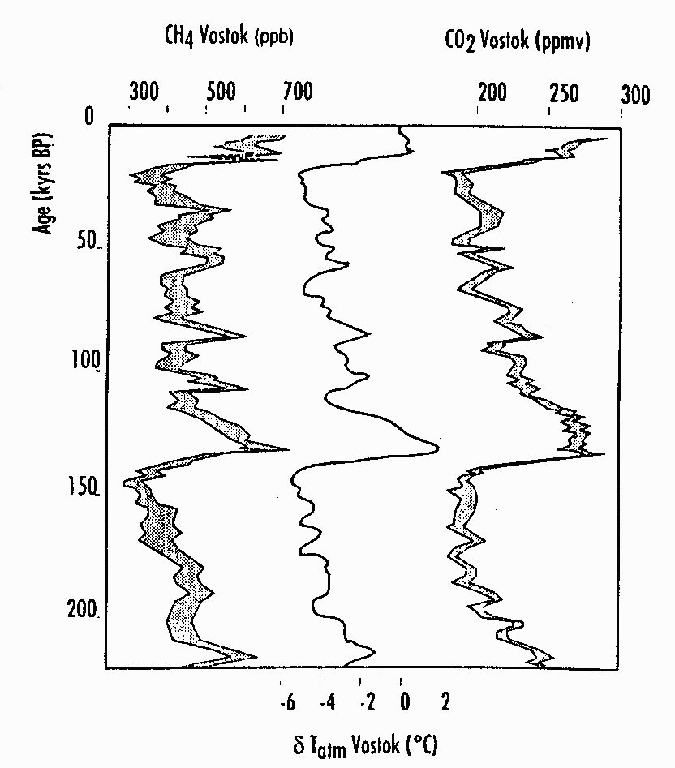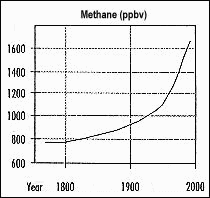Summer Research Program for Science Teachers

The Methane Project

Harvey Augenbran - Mott Hall School - 1997

Interactive Lesson:

The Role of the Atmosphere and Greenhouse Effect in Determining the Surface Temperature of the Earth

1. Background Information:

The surface temperature of the earth depends upon several factors. The dominant factor is the amount of solar energy arriving from the sun which depends on the earth's distance from the sun. The earth is about 150 million kilometers (km) [about 96 million miles] from the sun. We refer to this distance as 1 astronomical unit. When solar energy reaches the earth some of it is reflected by the atmosphere. The remainder passes through the atmosphere to the earth's surface where again some is reflected and some is absorbed. The reflected fraction of the total energy received is called the Albedo. This can be calculated at the top of the atmosphere or at the surface.

The surface of the earth absorbs solar energy, heats and then radiates energy, mostly as infra red, back out to space. Naturally occurring gases in the earth's atmosphere absorb some of the radiated infra red energy, heat and reradiate energy. Some of this reradiated energy is absorbed by the surface giving it additional energy. This process is the Natural Greenhouse Effect. [5-8 Content Standard D- Structure of earth system]

The earth's surface receives solar energy and energy reradiated by gases in the atmosphere. It heats and radiates energy back into space. The surface continues to heat until the amount of energy received at the is equal to the amount of energy radiated back into space. This condition is Radiative Balance. When this occurs the temperature is stable. [Content Standard Unifying Concepts- Equilibrium]

In this exercise, we will use interactive simulation courseware developed at NASA - GISS, and conduct research on greenhouse gases to explore the effect of an atmosphere and natural greenhouse gases on the surface temperature of the earth. [Content Standard Unifying Concepts- Evidence, models, and explanation] [Teaching Standard D- Make accessible technological resources] We will also look at natural and human caused (anthropogenic) perturbations in greenhouse gas concentrations and their possible affects on the surface temperature of the earth. [5-8 Content Standard F- Risks and benefits]

Below is a table for temperature conversion between Kelvin, Celsius and Fahrenheit.

 Kelvin = o Celsius = o Fahrenheit Kelvin = o Celsius = o Fahrenheit 250 -23 -9.4 273 0 +32.0 253 -20 -4.0 275 +2 +35.6 255 -18 -0.4 280 +7 +44.6 258 -15 +5.0 285 +12 +53.6 260 -13 +8.6 288 +15 +59.0 265 -8 +17.6 290 +17 +62.6 270 -3 +26.6

2. Surface Temperature - No Atmosphere

We will now look at what the earth's surface temperature would be if there were no atmosphere.

Begin the simulation program by following the prompts until you have "the earth with no atmosphere". Be sure to read the information in the dialog boxes as they appear.

What is the initial surface temperature? ________K = ______ o C = _______ o F

Describe what happens to solar energy after it reaches the surface.__________________________

______________________________________________________________________________

As you continue through the program, note the following information:

Describe what happened to the amount of radiated energy as the surface heated .

_______________________________________________________________________________

At the final step how many units of solar energy were available to be absorbed by the earth's

surface?________________

At the final step how many units of energy were radiated back? _______________

How do you know that radiative balance was reached? ( No, it isn't because it said so.)

_______________________________________________________________________________

What was the surface temperature when radiative balance was reached ?

________K = _______ o C = ______ o F

3a Surface Temperature - With an Atmosphere and No Greenhouse Gases

We will now investigate what the earth's surface temperature would be if we had an atmosphere without and then with greenhouse gases. Begin the simulation program by following the prompts until you have the "earth with an atmosphere but no greenhouse effect".

How many units of solar energy were available to be absorbed by the earth's surface?__________

How does this compare with the amount of solar energy that was available to be absorbed by the surface when there was no atmosphere?

_______________________________________________________________________________

_______________________________________________________________________________

What was the surface temperature when radiative balance was reached ?

________K = _______ o C = ______ o F

Compare the surface temperature reached at radiative balance when there was no atmosphere to the temperature when there was an atmosphere. Why were they different? [Teaching Standard B- Orchestrate scientific discourse]

_______________________________________________________________________________

_______________________________________________________________________________

3b. Surface Temperature - With an Atmosphere and Greenhouse Gases

Continue by adding natural greenhouse gases to the atmosphere.

How many units of solar energy were available to be absorbed by the earth's surface? ____________

How many units of energy are being radiated by the earth's surface? ________________

How could the amount energy being radiated by the surface be more than the amount of solar energy received?________________________________________________________________________

_______________________________________________________________________________

What was the surface temperature when radiative balance was reached?

________K = _______ o C = _______ o F

4. Natural Changes in Concentrations of Greenhouse GasesThe previous exercises showed that the greenhouse effect occurs naturally and actually is beneficial. On the earth, water vapor (H2O), carbon dioxide (CO2), methane (CH4) and nitrous oxide (N2O) are the gases most responsible for this effect.

The graph on left (figure 1) shows concentrations of atmospheric methane and carbon dioxide in the atmosphere, as well as temperature changes that occurred over the last 200,000 years. The data were obtained from examining gases trapped in ice cores from Vostok, Antarctica. [5-8 Content Standard D- Earth's history] The y-axis (kyrs BP) shows the time in thousands of years before the present. The x-axis on top shows the concentration of methane in parts per billion in a given volume (left) and the concentration of carbon dioxide in parts per million in a given volume (right). The x-axis on the bottom (center) shows variations around the mean temperature over this time. Notice that even during periods where concentrations are generally decreasing, there are oscillations to higher and lower values. These are natural variations in the concentration of greenhouse gases.

What is the time period covered by the graph?

 CO2 CH4 Temperature What is the maximum concentration of CO2 & CH4 and temperature 140,000 years ago? (this is our initial value) ppmv ppbv OC What is the minimum concentration of CO2 & CH4 and temperature 20,000 years ago? (this is our final value) ppmv ppbv OC What was the change in CO2 & CH4 and temperature for this period? ppmv ppbv OC Did they increase or decrease? Calculate the percentage change for CO2 & CH4. % Change = Change Initial Value

We can now use the simulation program to see what temperature changes might occur with the change in concentrations of CO2 and CH4 that we calculated from the ice core data.

Go to the program and follow the prompts until you get to the "change greenhouse gases" dialog box. Choose CO2 first and change the percentage to the value you calculated.

What is the change in temperature? ________K = _______ o C = _______ o F

Repeat the procedure for CH4.

What is the change in temperature? ________K = _______ o C = _______ o F

How does the combined temperature change shown by the program compare to the change given in the graph? ______________________________________________________________________

Why do you think this happened?

[5-8 Content Standard A- Use appropriate tools AND use evidence to explain; recognize alternative explanations]

______________________________________________________________________________

(discuss this question because there are many factors involved here)

5. Anthropogenic Changes in Concentrations of Greenhouse GasesSince the beginning of the Industrial Revolution about 200 years ago, human activities (anthropogenic) have been influencing the concentration of greenhouse gases. Examine the graph on the right (Figure 2). This graph shows the atmospheric concentration of CO2 for the past 200 years. This type of graph shows concentrations as well as trends over time. The y-axis shows concentration of CO2 in parts per million in a given volume - ppmv . The x-axis shows the years the measurements were taken. Note that the data line is not straight. This means that the rate of change is not constant for the entire time.

What is the approximate change in concentration of CO2 from 1800 to 1900? _________________ppmv

What is the approximate change in concentration of CO2 from 1900 to 1990?__________________ppmv

Calculate the percentage change in CO2 concentration from 1800 to 1990 ___________________

Put this value into the simulation program.

What is the resulting change in temperature? ? ________K = _______ o C = _______ o FExamine the graph on the left (Figure 3). This graph shows the atmospheric concentration of CH4 for the past 200 years. The y-axis shows concentration of CH4 in parts per billion in a given volume - ppbv. The x-axis shows the years the measurements were taken.

Is the change in methane concentration constant for the entire time period shown? _____________________

Calculate the percentage change in CH4 concentration for 1800 to 1990. ______________________

Put this value into the simulation program. What was the resulting change in temperature?Examine the graph on the right (Figure 4). This graph shows the atmospheric concentration of Nitrous Oxide (N2O) in parts per billion for a given volume for the past 200 years.

Using the graph, determine when the greatest rate of change occurred? _______________________

Calculate the percentage change in N2O concentration from 1800 to 1900. _______________________

Put this value into the simulation program. What was the resulting change in temperature?

6. Critical Thinking

Using the information you derived from the 3 graphs, what pattern seems to emerge regarding the rate at which the concentration of these greenhouse gases are increasing? [5-8 Content Standard A- Summarize data; argue cause and effect}

_____________________________________________________________________________________________

What prediction could you make if these trends were to continue?________________________________________

_____________________________________________________________________________________________